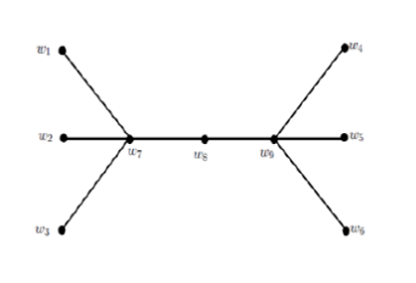• P-ISSN 0974-6846 E-ISSN 0974-5645# Indian Journal of Science and Technology

## Article• VIEWS 1392
• PDF 204

Indian Journal of Science and Technology

Year: 2022, Volume: 15, Issue: 20, Pages: 976-982

Original Article

## Gamma coloring of Mycielskian graphs

Received Date:11 December 2021, Accepted Date:04 February 2022, Published Date:28 May 2022

## Abstract

Background: Given a graph G, the gamma coloring problem seeks for a proper coloring C of G with the property that there exists a dominating set of G in which all the vertices receive different colors under the coloring C. The minimum number of colors required for a gamma coloring of G is called the gamma chromatic number of G and is denoted by cg (G). Our aim is to find the gamma chromatic number of Mycielskian graphs. Methods: Here, we obtain gamma coloring for Mycielskian graph m(G) from a gamma coloring of G by generalizing the give gamma coloring of G. To prove cg (m(G))  m for a graph G, we gave a gamma coloring to m(G) using m colors. To prove cg (m(G)) = m for a graph G, we first proved that cg (m(G))  m and then gave a gamma coloring to m(G) using m colors. Finding: In this paper, we have initiated a study on Gamma coloring for Mycielskian graph m(G) of a given graph G. We have proved that, the gamma chromatic number cg for m(G) is either cg (G) or cg (G)+1 and thus, we classify the class of all connected graphs into two classes namely Class-1 and Class-2 graphs. Graphs G for which cg (m (G)) =cg (G) are of Class-1 and rest of the graphs are of Class-2. Conditions under which a graph G becomes Class-1/ Class-2 have been established. Novelty: One can investigate towards finding a structural characterization of graph G with cg (m (G)) = cg (G) or cg (m (G)) = cg (G)+1. Gamma coloring is a new variation of graph coloring in which the concepts of coloring and domination are linked using the condition that the coloring admits a dominating set in which every vertex receives different colors and, in this paper, we study about the gamma coloring of Mycielskian graph m(G) of a graph G.

Keywords: Coloring; Dominating Set; Colorful Set; Mycielskian Graphs; Gamma Coloring

## References

1. Abid A, Mohammed TR, Rao. Dominator coloring of Mycielskian graphs. The Australasian Journal of Combinatorics. 2019;73:274–279. Available from: https://ajc.maths.uq.edu.au/pdf/73/ajc_v73_p274.pdf
2. Abid AM, Rao TRR. On strict strong coloring of graphs. Discrete Mathematics, Algorithms and Applications. 2021;13(04):2150040. Available from: https://dx.doi.org/10.1142/s1793830921500403
3. Balakrishnan R, Raj SF. Connectivity of the Mycielskian of a graph. Discrete Mathematics. 2008;308(12).
4. Guo L, Liu R, Guo X. Super Connectivity and Super Edge Connectivity of the Mycielskian of a Graph. Graphs and Combinatorics. 2012;28(2):143–147. Available from: https://dx.doi.org/10.1007/s00373-011-1032-3
5. Bidine EZ, Gadi T, Kchikech M. Independence number and packing coloring of generalized Mycielski graphs. Discussiones Mathematicae Graph Theory. 2021;41(3):725. Available from: https://dx.doi.org/10.7151/dmgt.2337
6. Chen M, Guo X, Li H, Zhang L. Total chromatic number of generalized Mycielski graphs. Discrete Mathematics. 2014;334:48–51. Available from: https://dx.doi.org/10.1016/j.disc.2014.06.010
7. Savitha KS, Chithra MR, Vijayakumar A. Some Diameter Notions of the Generalized Mycielskian of a Graph. In: Theoretical Computer Science and Discrete Mathematics. (pp. 371-382) Springer International Publishing. 2017.
8. Tang Y, Zhu X. Total weight choosability of Mycielski graphs. Journal of Combinatorial Optimization. 2017;33(1):165–182. Available from: https://doi.org/10.1007/s10878-015-9943-1
9. Shen Y, An X, Wu B. Hamilton-Connected Mycielski Graphs∗. Discrete Dynamics in Nature and Society. 2021;2021:1–7. Available from: https://dx.doi.org/10.1155/2021/3376981
10. Zhong Y, Hayat S, Khan A. Hamilton-connectivity of line graphs with application to their detour index. Journal of Applied Mathematics and Computing. 2022;68(2):1193–1226. Available from: https://dx.doi.org/10.1007/s12190-021-01565-2
11. Fisher DC, McKenna PA, Boyer ED. Hamiltonicity, diameter, domination, packing, and biclique partitions of Mycielski's graphs. Discrete Applied Mathematics. 1998;84(1-3):93–105. Available from: https://dx.doi.org/10.1016/s0166-218x(97)00126-1
12. Larsen M, Propp J, Ullman D. The fractional chromatic number of a graph and a construction of Mycielski. Journel of Graph theory. 1995;19:411–416. Available from: https://doi.org/10.1002/jgt.3190190313Article Metrics